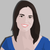# Calculate value python 3

538
3
05-28-2020 05:01 AMOccasional Contributor

Hi all

Am struggling to work out how to update a value for an integer attribute (SLD) that is based on the values in 3 other integer fields "P", "O", "S".

The following is the code I could use in ArcGIS for Desktop 10.7.1 calculate field (and based on VB):

Dim x
if

= 1 AND = 1 AND = 1 Then
x = 1
elseIf

= 1 AND = 1 AND = 2 Then
x = 2
elseIf

= 1 AND = 1 AND = 3 Then
x = 3
elseIf

= 1 AND = 2 AND <= 4 Then
x = 4
elseIf

= 1 AND = 3 AND <> 4 Then
x = 5
elseIf

= 1 AND = 5 AND <= 3 Then
x = 6
elseIf

= 4 AND <> 4 AND <= 5 Then

x = 7

else
x = 0

end if

If anyone can provide me with some suggestions as to how to convert this into python 3 within ArcGIS Pro I would be most appreciative,

Many thanks,

Mark

1 Solution

Accepted SolutionsbyMVP Regular Contributor

Hi Mark,

This should get you what you need:

``````def fieldCalc(f1, f2, f3):
x = 0
if f1 == 1 and f2 == 1 and f3 == 1:
x = 1
elif f1 == 1 and f2 == 1 and f3 == 2:
x = 2
elif f1 == 1 and f2 == 1 and f3 == 3:
x = 3
elif f1 == 1 and f2 == 2 and f3 <= 4:
x = 4
elif f1 == 1 and f2 == 3 and f3 != 4:
x = 5
elif f1 == 1 and f2 == 5 and f3 <= 3:
x = 6
elif f1 == 4 and f2 != 4 and f3 <= 5:
x = 7
return x‍‍‍‍‍‍‍‍‍‍‍‍‍‍‍‍‍‍‍‍‍‍‍‍‍‍‍‍‍‍‍‍‍‍‍‍‍‍‍‍‍‍‍‍‍‍‍‍‍‍‍‍‍‍‍‍‍‍‍‍‍‍‍‍‍‍‍‍‍‍‍‍``````

The above code would be put in the Code Block, and you would call the function with SLD = fieldCalc(!P!, !O!, !S!)

Some of the major differences between VB Script and Python in relation to this code segment:

• Field names are referenced by surrounding the name with ! instead of [ ]
• The "and" operator is lowercase in Python
• If statements end with a colon instead of the word "THEN"
• In Python, the == means "is equivalent to", whereas the single = is used to assign a value to a variable
• In Python, use != for "does not equal" rather than <>
• In Python, use "elif" instead of "elseif"
• Don't forget the return statement at the end!

Luckily, there are countless resources online where you can get even more information about operators and logical statements in Python.

There is likely an even fancier way to do this, but my version should work and it was the simplest adaptation from your code.

Hope that helps!-Katherine

Best,
Katherine
3 RepliesbyMVP Regular Contributor

Hi Mark,

This should get you what you need:

``````def fieldCalc(f1, f2, f3):
x = 0
if f1 == 1 and f2 == 1 and f3 == 1:
x = 1
elif f1 == 1 and f2 == 1 and f3 == 2:
x = 2
elif f1 == 1 and f2 == 1 and f3 == 3:
x = 3
elif f1 == 1 and f2 == 2 and f3 <= 4:
x = 4
elif f1 == 1 and f2 == 3 and f3 != 4:
x = 5
elif f1 == 1 and f2 == 5 and f3 <= 3:
x = 6
elif f1 == 4 and f2 != 4 and f3 <= 5:
x = 7
return x‍‍‍‍‍‍‍‍‍‍‍‍‍‍‍‍‍‍‍‍‍‍‍‍‍‍‍‍‍‍‍‍‍‍‍‍‍‍‍‍‍‍‍‍‍‍‍‍‍‍‍‍‍‍‍‍‍‍‍‍‍‍‍‍‍‍‍‍‍‍‍‍``````

The above code would be put in the Code Block, and you would call the function with SLD = fieldCalc(!P!, !O!, !S!)

Some of the major differences between VB Script and Python in relation to this code segment:

• Field names are referenced by surrounding the name with ! instead of [ ]
• The "and" operator is lowercase in Python
• If statements end with a colon instead of the word "THEN"
• In Python, the == means "is equivalent to", whereas the single = is used to assign a value to a variable
• In Python, use != for "does not equal" rather than <>
• In Python, use "elif" instead of "elseif"
• Don't forget the return statement at the end!

Luckily, there are countless resources online where you can get even more information about operators and logical statements in Python.

There is likely an even fancier way to do this, but my version should work and it was the simplest adaptation from your code.

Hope that helps!-Katherine

Best,
KatherineOccasional Contributor

Thank you so much Katherine,

This works brilliantly and easy to understand and has saved me a fair few hours! Undoubtedly there may be other methods, but will stick with easy for now.

Once again, thank you!

MarkbyMVP Regular Contributor

So glad I could help!Best,
Katherine